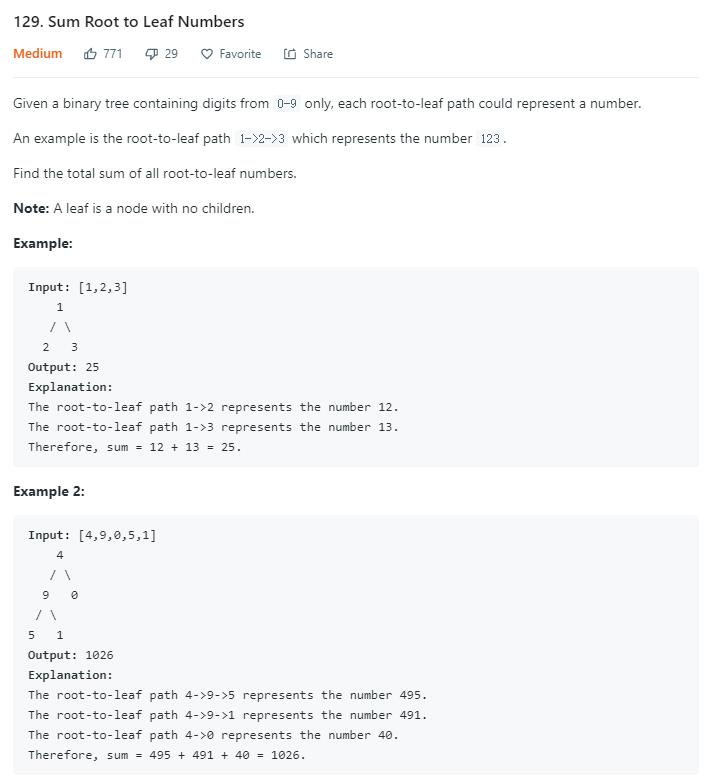# 题目描述（中等难度）# 思路分析

112 题 有些像，112 题是给出一个 sum，然后去找这条路径。但本质上都一样的，只需要对二叉树进行遍历，遍历过程中记录当前路径的和就可以。说到遍历，无非就是 BFSDFS，如果进行 BFS，过程中我们需要维护多条路径的和，所以我们选择 DFS

# 解法一 回溯法

void dfs(TreeNode root, int cursum)


private void dfs(TreeNode root, int cursum) {
if (root.left == null && root.right == null) {
sum += cursum;
return;
}


public int sumNumbers(TreeNode root) {
if (root == null) {
return 0;
}
dfs(root, root.val);
return sum;
}

int sum = 0;

private void dfs(TreeNode root, int cursum) {
//到达叶子节点
if (root.left == null && root.right == null) {
sum += cursum;
return;
}
//尝试左子树
if(root.left!=null){
dfs(root.left,  cursum * 10 + root.left.val);
}
//尝试右子树
if(root.right!=null){
dfs(root.right, cursum * 10 + root.right.val);

}

}


# 解法二 分治法

int sumNumbersHelper(TreeNode root, int sum)


int cursum = sum * 10 + root.val;


int ans1 = sumNumbersHelper(root.left,cursum)


int ans2 = sumNumbersHelper(root.right,cursum)


public int sumNumbers(TreeNode root) {
if (root == null) {
return 0;
}
return sumNumbersHelper(root, 0);
}

private int sumNumbersHelper(TreeNode root, int sum) {
//已经累计的和
int cursum = sum * 10 + root.val;
if (root.left == null && root.right == null) {
return cursum;
}
int ans = 0;
//从最开始经过当前 root 再经过左孩子到达叶子节点所有的路径和
if (root.left != null) {
ans += sumNumbersHelper(root.left, cursum);
}
//从最开始经过当前 root 再经过右孩子到达叶子节点所有的路径和
if (root.right != null) {
ans += sumNumbersHelper(root.right, cursum);
}
//返回从最开始经过当前 root 然后到达叶子节点所有的路径和
return ans;
}